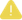# Continue If

Continue or halt the flow based on the result of a true or false conditional test. Use Return Error If instead if you want to halt the flow with an error when the condition is true.

## Input

Field Definition Type Required
value a Value to be compared. Various FALSE
comparison Comparison to make (see table below). Various FALSE
value b Value to compare against. Various FALSE
message An optional value to return when the condition is false and the flow stops. Various FALSEBe sure to set the type of value a and value b to reflect how you would like the comparison to be done. For instance, the operator "greater than" compares numbers based on numeric value but text based on alphabetical sort order. So the number comparison 80 > 9 is true, but the text comparison "80" > "9" is false.

## Output

Field Definition Type
message An optional value to return when the condition is false and the flow stops. String

## Table of Relational Operators

Operator

Use Case

equal to Compare two texts, numbers, dates or true/false to see if they have the same value.
not equal to Compare two texts, numbers, dates or true/false to see if they have different values.
greater than or equal Compare two texts, numbers or dates to see if the first has the same or greater value than the second.
less than or equal Compare two texts, numbers or dates to see if the first has the same or lesser value than the second.
greater than Compare two texts, numbers or dates to see if the first has a greater value than the second.
less than Compare two texts, numbers or dates to see if the first has a lesser value than the second.
a multiple of Compare two numbers to see if value a is an exact multiple of value b. For example, 12, 0 and -4 are all multiples of 4, but 2, 3 and 7 are not.
in Compare two texts to see if value a is a part of value b. For example, "test" is in "this is a test", but there is no "i" in "team".
not in Compare two texts to see if value a is not part of value b.
has key When value a is an object and value b is text, tests to see if the object has a key with the specified name. For example, true if value a is {"test":123} and value b is "test".
doesn't have key When value a is an object and value b is text, tests to see if the object does not have a key with the specified name.
is empty Checks to see if value a is empty; ignores value b. What defines "empty" varies by type. Note: Only works for Text and Object. Numbers, Dates, T/F, are viewed as empty.
is not empty Checks to see if value a is not empty. Note: Only works for Text and Object. Numbers, Dates, T/F, are viewed as empty.